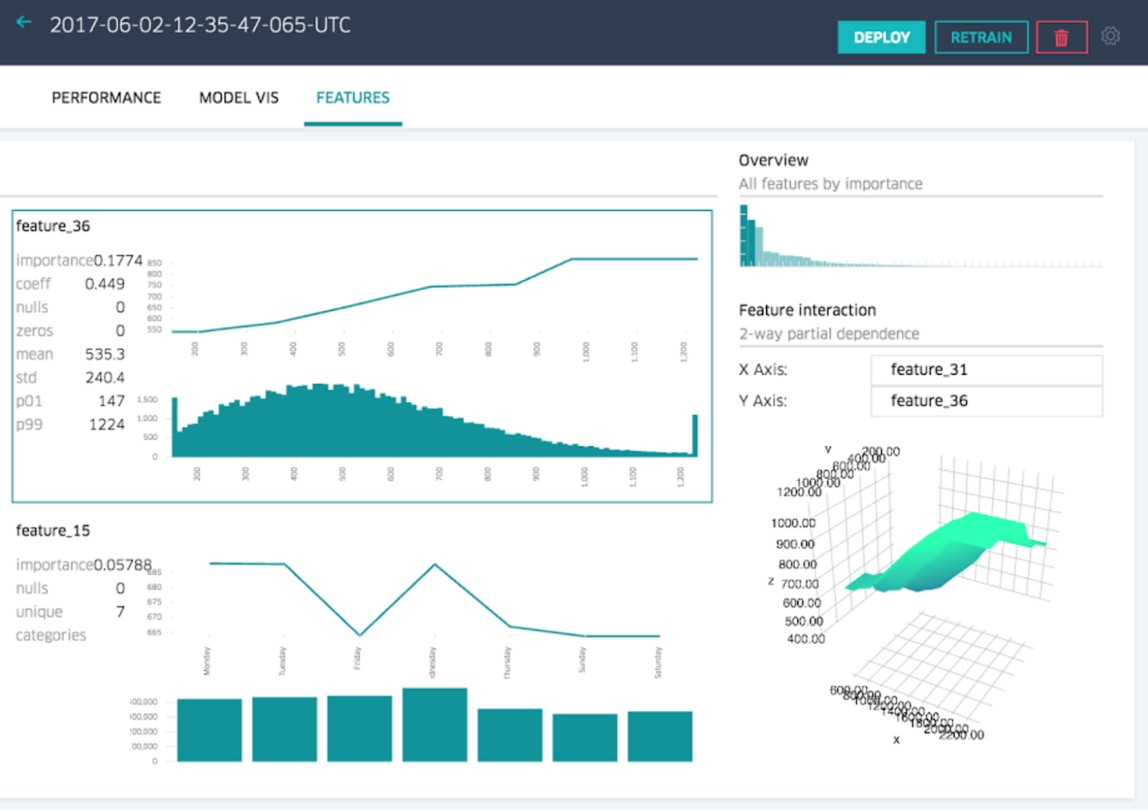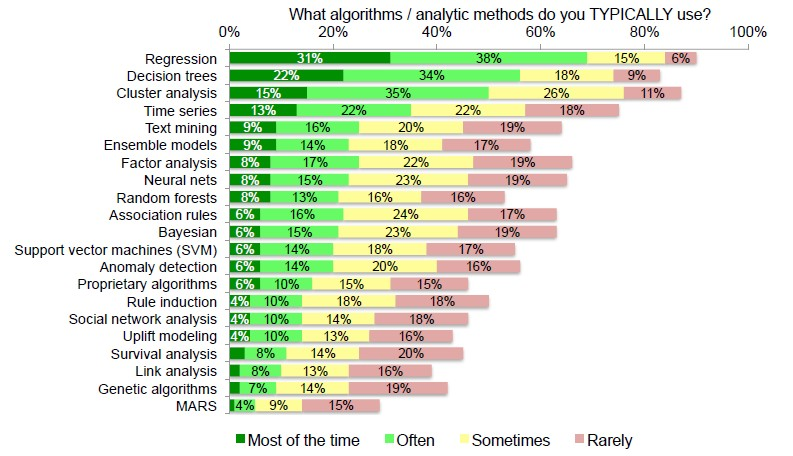# Statistics - Generalized Linear Models (GLM) - Extensions of the Linear Model

The Generalized Linear Model is an extension of the linear model that allows for lots of different, non-linear models to be tested in the context of regression.

GLM is the mathematical framework used in many statistical analyses such as:

GLM is a supervised algorithm with a classic statistical technique (Supports thousands of input variables, text and transactional data) used for:

GLM implements:

Confidence bounds are supported with a

• GLM classification for prediction probabilities.
• GLM regression for predictions.

## Assumptions

The General Linear model has two main characteristics:

• Linear: linear relationships between the predictors and the outcome measure.
• Additive: the effects of each predictor are additive with one another

That doesn't mean that the GLM can't handle non-additive or non-linear effects.

GLM can accommodate such non-additive or non-linear effects with:

• Transformation of variables: in order to make them linear
• Adding interaction terms or moderation terms: in order to do a moderation analysis and test for non-additive facts.

## Methods

Methods that expand the scope of linear models and how they are fit:

• Classification problems: logistic regression, support vector machines
• Non-linearity: kernel smoothing, splines and generalized additive models; nearest neighbour methods.
• Interactions: Tree-based methods, bagging, random forests and boosting (these also capture non-linearities)
• Regularized fitting: Ridge regression and lasso. These have become very popular lately, especially when we have data sets where we have very large numbers of variables–so-called wide data sets, and even linear models are too rich for them, and so we need to use methods to control the variability.

Recommended PagesData Mining - (Attribute|Feature) (Selection|Importance)

Feature selection is the second class of dimension reduction methods. They are used to reduce the number of predictors used by a model by selecting the best d predictors among the original p predictors....Data Mining - (Dimension|Feature) (Reduction)

In machine learning and statistics, dimensionality reduction is the process of reducing the number of random variables (features) under consideration and can be divided into: feature selection (returns...Data Mining - Algorithms

An is a mathematical procedure for solving a specific kind of problem. For some data mining functions, you can choose among several algorithms. Algorithm Function Type Description Decision...ORE (Oracle R Enterprise)

Oracle R Enterprise is a component of Oracle...R

is a language and environment for statistical computing and graphics. Syntax is very similar to the S_(programming_language)S language. S is a language that was developed by John Chambers et al. at Bell...R - Generalized linear model (glm)

glm glm with the argument family equals binomial in order to apply the logit transformation. contingency tables or class tabs.R - Glm Function

Glm can fit linear and non-linear models in particular logistic regression models.Statistics - Binary logistic regression

logistic regression for a binary outcome. where: : predicted value on the outcome variable Y : the outcome variable : predicted value on Y when all X = 0 : predictor variables : unstandardized...Statistics - Centering Continous Predictors

By putting all scores of a variable in a deviation form, the average for this variable will be equal to zero. It's called centering. To center means to put in deviation form. To center a variable, take...Statistics - Dummy (Coding|Variable) - One-hot-encoding (OHE)

Dummy coding is: a classic way to transform nominal into numerical values. a system to code categorical predictors in a regression analysis A system to code categorical predictors in a regression...